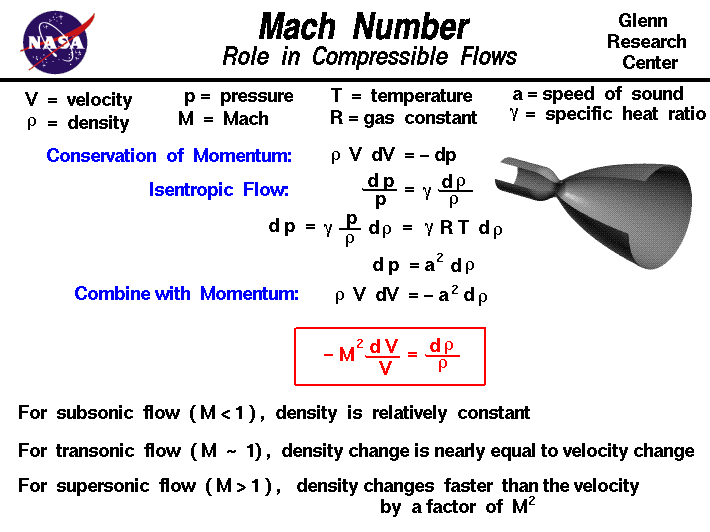+ Text Only Site
+ Non-Flash Version
+ Contact GlennAs an aircraft moves through the air, the air molecules near the aircraft are disturbed and move around the aircraft. If the aircraft passes at a low speed, typically less than 250 mph, it is observed that the density of the air remains constant. For higher speeds, some of the energy of the aircraft goes into compressing the air and locally changing the density of the air. This compressibility effect alters the amount of resulting force on the aircraft since the aerodynamic force depends on the air density. The effect becomes more important as speed increases. Near and beyond the speed of sound, about 330 m/s or 760 mph at sea level, small disturbances in the flow are transmitted to other locations isentropically or with constant entropy. Sharp disturbances generate shock waves that affect both the lift and drag of the aircraft, and the flow conditions downstream of the shock wave. On this slide, we will investigate the dependence of the density change on the Mach number of the flow. The Mach number is the ratio of the speed of the aircraft, or the speed of the gas, to the speed of sound in the gas. The speed of sound is equal to the speed of transmission of small, isentropic disturbances in the flow. To determine the role of the Mach number on compressibility effects. we begin with the conservation of momentum equation: rho * V dV = - dp where rho is the fluid density, V is the velocity, and p is the pressure. dV and dp denote differential changes in the velocity and pressure. From our derivation of the conditions for isentropic flow, we know that: dp/p = gamma * drho/rho dp = gamma * p / rho * drho where gamma is the specific heat ratio. We can use the ideal equation of state to simplify the expression on the right: p = rho * R * T dp = gamma * R * T * drho where R is the specific gas constant and T is the absolute temperature. We recognize that: gamma * R * T = a^2 where a is equal to the speed of sound. So, dp = a^2 * drho Substituting this expression for the change of pressure into the conservation of momentum equation gives: rho * V dV = - a^2 drho - (V^2 / a^2) dV / V = drho / rho - M^2 dV / V = drho / rho where M is the Mach number. What does this expression tell us about the role of the Mach number in compressible flows? For low speed, or subsonic conditions, the Mach number is less than one, M < 1 and the square of the Mach number is very small. Then the left hand side of the equation is very small, and the change in density is very small. For the low subsonic conditions, compressibility can be ignored. As the speed of the object approaches the speed of sound, the flight Mach number is nearly equal to one, M = 1, and the flow is said to be transonic. If the Mach number is near one, the square of the Mach number is also nearly equal to one. For transonic flows, the change in density is nearly equal to the change in velocity, and compressibility effects can not be ignored. As the speed increases beyond the speed of sound, the flight Mach number is greater than one M > 1 and the flow is said to be supersonic or hypersonic. For supersonic and hypersonic flows, the density changes faster than the velocity changes by a factor equl to the square of the Mach number. Compressibility effects become more important with higher Mach numbers. Activities: Guided Tours Navigation ..Beginner's Guide Home Page+ Inspector General Hotline + Equal Employment Opportunity Data Posted Pursuant to the No Fear Act + Budgets, Strategic Plans and Accountability Reports + Freedom of Information Act + The President's Management Agenda + NASA Privacy Statement, Disclaimer, and Accessibility CertificationEditor: Nancy Hall NASA Official: Nancy Hall Last Updated: May 05 2015 + Contact Glenn